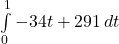## A ball is launched straight up in the air from a height of 6 feet. Its velocity​ (feet/second) t seconds after launch is given by f(t)= -34t

Question

A ball is launched straight up in the air from a height of 6 feet. Its velocity​ (feet/second) t seconds after launch is given by f(t)= -34t+291.
Between 1 second and 8 seconds, the​ ball’s height changed by … feet.
​(Round answer to the nearest​ tenth.)

in progress 0
2 weeks 2021-09-02T05:39:59+00:00 1 Answers 0 views 0

966 feet

Step-by-step explanation:

First, we can see that we have the velocity function given, a base value for the height, and need to figure out the change in height. We also know that velocity is the derivative of position/height. Thus, we can find the integral of the velocity to find an equation for the height.Use the exponent rule to turn -34t into -17t² and 291 into 291t to get our result as

-17t²+291t + C = height (h)

When t=0, we know that our height (h) is 6, so C = 6, making our equation

-17t²+291t + 6

To find the change between 1 second and 8 seconds, we can plug 1 and 8 in for t, and find the difference between those values, which is

(-17(8)²+291(8) + 6) – ( -17(1)²+291(1) + 6 )

= 1246 – 280

= 966

Note that we did final value (t = 8) – initial value (t=1), not the other way around

The change in height, between 1 second and 8 seconds, is therefore 966 feet